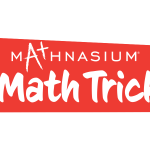Get them the math skills they need
to have a great school year.

Welcome to Mathnasium—your neighborhood math-only learning center that teaches kids math the way that makes sense to them. Our experienced math tutors utilize our proprietary teaching materials and techniques, The Mathnasium Method™, to deliver a customized learning plan designed to address each student's needs, whether they started out far behind or are already ahead in math. Our instruction approach goes beyond traditional math tutoring to develop understanding and build a love for math.

20 October 2021Here is a number sense trick to help you calculate multiplication using mental math.

We want to find the product of 5 x 4 x 3 x 2 x 1. Using our number sense, we want to find numbers close to ten or multiples of ten to make the multiplication easier.

18 October 2021Here is a number sense trick to help you calculate subtraction using mental math.

We want to find the difference of 100 – 76. Using our number sense, we turn to “friendly numbers” to calculate how far apart are 76 and 100.

Starting at 76, we can first find how far apart are 76 and 80 (the nearest multiple of 10) and get 4.

13 October 2021We want to find the sum of 9 + 8 + 7. Using our number sense, we turn to “friendly numbers” such as 10 or multiples of 10, to help make this math easier to work with.

29 Jun'20

LOS ANGELES, June 29, 2020 /PRNewswire/ -- Mathnasium Learning Centers — com...

7 May'20

Mathnasium is a proud founding sponsor of National PTA's STEM + Families program, which award...

23 Apr'20

LOS ANGELES, April 23, 2020 /PRNewswire/ -- Mathnasium Learning Centers, LLC recen...